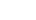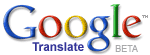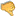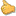ترجم الموضوع الى العربيةA Mathematical Theory of JusticeHassan Ajami
2019 / 9 / 30

Philosophical theories could be successfully presented in mathematical equations. This is the mathematization of philosophy. For example, we could mathematically analyze justice in the following way: justice = peace x freedom x equality. In this mathematical theory of justice, justice is analyzed in terms of peace, freedom, and equality, and as being a mathematical relationship and/or equation, such that justice is equal to peace multiplied by freedom which is in turn multiplied by equality. This mathematical equation of justice implies that the best kind of justice�-or-the maximum amount of justice is obtained if and only if we have maximum amount�-or-degree of peace, freedom, and equality for each and every person.

It follows from this mathematical equation of justice that there are different kinds of justice. Since justice = peace x freedom x equality, it mathematically follows that peace is equal to justice, such that justice is divided by the multiplication of freedom and equality. And thus, justice could be maintained when peace is established even if freedom and equality are minimum but not equal to zero (given that whatever is divided by zero is indefinite). This is the first kind of justice, according to which, justice exists due to the existence of peace. And it is justice as peace.

Moreover, since justice = peace x freedom x equality, it also mathematically follows that freedom is equal to justice, such that justice is divided by the multiplication of peace and equality. Thus, justice could be achieved when freedom is maintained and maximized, even if peace and equality are minimum yet not equal to zero (given that whatever is divided by zero is indefinite). This would be justice as freedom. And it is the second kind of justice, according to which, justice consists of freedom and the maximization of freedom, regardless of the maximization of peace and equality.

A third kind of justice follows from the same equation. Since justice = peace x freedom x equality, it mathematically follows that equality is equal to justice, such that justice is divided by the multiplication of peace and freedom. Therefore, justice is maintained when equality is established and maximized, even if peace and freedom are minimum but not equal to zero (given that whatever is divided by zero is indefinite). This is justice as equality. And it is the third kind of justice. Equality, in this context, could be understood as equality before the law, equality of opportunity, and/or social and economic equality. Yet the best kind of justice is the fourth kind, according to which, justice is maintained if and only if peace, freedom, and equality are maximized at the same time, given that justice = peace x freedom x equality.

In addition, this mathematical theory of justice is successful in accounting for the fact that justice is a matter of degree, leading to the conclusion that it is a successful and accurate theory. Given that justice = peace x freedom x equality, it follows that justice is maximized when peace, freedom, and equality are maximum, while justice is minimized when peace, freedom, and equality are minimized. Thus, justice is a matter of degree, such that we could have more�-or-less justice. All of this shows that the mathematical theory of justice entails that justice is a matter of degree, and hence explains why it is a matter of degree. In other words, justice is a matter of degree because justice = peace x freedom x equality which could be maximized�-or-minimized.

The mathematical theory of justice analyzes justice in terms of peace because without peace there will be neither freedom nor equality. Therefore, justice could not be maintained without peace. People living in war zones are either killed�-or-wounded,�-or-illegally imprisoned�-or-kidnapped, and their different kinds of freedom, such as the freedom of speech and movement, are violated due to the atrocities of war which violate human rights. This is why justice as freedom could not be obtained in the time of war. And hence, peace is the first condition which should be satisfied in order to have justice as freedom.

Similarly, in wartime, people are not treated equally, given that war violates the human rights of some people through the killings and wounding, and oppressing some and illegally imprisoning�-or-kidnapping others. This shows that peace should be established and protected in order to have justice as equality. In light of these considerations, peace should be considered to be the first principle of justice in order to maintain any sort of justice, such as justice as freedom�-or-justice as equality. And this is why the mathematical theory of justice defines justice in terms of peace in addition to defining justice in terms of freedom and equality. Justice without peace is senseless, while justice without freedom and equality is incomplete.Bad 12345678910 Very good